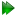Subsections

Rotor equations

The only motion is in the torsion angle about the rotation axis.

Figure 16.1: The rotor model simulated and calculated in-frame Daeg(1) and Daeg(2) frame order matrix elements. The top row corresponds to Daeg(1) and the bottom to Daeg(2). In these plots, θZ corresponds to the torsion half-angle σmax. Frame order matrix values have been calculated every 10 degrees.Figure 16.2: The rotor model simulated and calculated out-of-frame Daeg(1) and Daeg(2) frame order matrix elements. The top row corresponds to Daeg(1) and the bottom to Daeg(2). In these plots, θZ corresponds to the torsion half-angle σmax. Frame order matrix values have been calculated every 10 degrees.Rotor rotation matrices

Assuming the rotation axis is the z-axis, the rotation matrix is defined as

 R(σ) =. (16.10)

Rotor frame order matrix

The frame order matrix isThe surface normalisation factor isRotor 1 degree frame order

The 1 degree frame order matrix with tensor rank-2 isRotor 2 degree frame order

The 2 degree frame order matrix with tensor rank-4 iswhere sσ = sincσmax and s2σ = sinc(2σmax). The active matrix elements which are not zero due to symmetries, in Kronecker product double indices from 0 to 8, areRotor frame order matrix simulation and calculation

The frame order matrix element simulation script from Section 16.2, pagewas used to compare the implementation of equations 16.13 and 16.15 above. Frame order matrix Daeg(1) and Daeg(2) values were both simulated and calculated, both within and out of the motional eigenframe. The in-frame Daeg(1) and Daeg(2) values are shown in figure 16.1. The out-of-frame Daeg(1) and Daeg(2) values are shown in figure 16.2.

The relax user manual (PDF), created 2020-08-26.